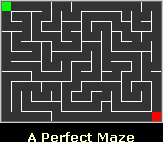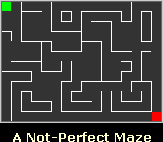# Step by step perfect maze generation with php

This is a project I made for teaching purpose.
First of all, let’s see what is a perfect maze.
From Maze Works: A perfect maze is defined as a maze which has one and only one path from any point in the maze to any other point. This means that the maze has no inaccessible sections, no circular paths, no open areas.

This is a perfect mazeThis is not a perfect mazeWith the script I provide, you can generate perfect mazes with a given length and height, and the cute thing is that the script will “think loud” its actions in order to explain how does it work.
It uses backtracking.

The program, like most maze generators, starts off by building a maze with all the walls between the cells intact.
Then chooses a random cell where to start and mark the current cell as ‘Visited’;

If the current cell has any neighbour cells which have not been visited

• Randomly choose one of these cells
• Knock down the wall between current and choosen cells
• Make the chosen cell the current cell
• Mark the new current cell as visited

else

• Go to your previoulsy visited cell

Until all cells are visited

So, you can use it both for generating perfect mazes, and to show how would you generated a perfect maze.

In the example, the script executed with a 5×5 maze.

Later I will explain the code, meanwhile here it is:

```";

\$maze[\$pos]{0} = 1;
\$visited ++;

// determine possible directions
while(\$visited<\$cell_count){
\$possible = "";
if((floor(\$pos/\$dim_x)==floor((\$pos-1)/\$dim_x)) and (\$maze[\$pos-1]{0}==0)){
\$possible .= "W";
}
if((floor(\$pos/\$dim_x)==floor((\$pos+1)/\$dim_x)) and (\$maze[\$pos+1]{0}==0)){
\$possible .= "E";
}
if(((\$pos+\$dim_x)<\$cell_count) and (\$maze[\$pos+\$dim_x]{0}==0)){
\$possible .= "S";
}
if(((\$pos-\$dim_x)>=0) and (\$maze[\$pos-\$dim_x]{0}==0)){
\$possible .= "N";
}
\$html .= "I am in \$pos and I can go to: \$possible";
if(\$possible){
\$visited ++;
array_push(\$moves,\$pos);
\$direction = \$possible{rand(0,strlen(\$possible)-1)};
\$html .= "I randomly choose to go \$direction";
switch(\$direction){
case "N":
\$maze[\$pos]{1} = 0;
\$maze[\$pos-\$dim_x]{2} = 0;
\$pos -= \$dim_x;
break;
case "S":
\$maze[\$pos]{2} = 0;
\$maze[\$pos+\$dim_x]{1} = 0;
\$pos += \$dim_x;
break;
case "E":
\$maze[\$pos]{3} = 0;
\$maze[\$pos+1]{4} = 0;
\$pos ++;
break;
case "W":
\$maze[\$pos]{4} = 0;
\$maze[\$pos-1]{3} = 0;
\$pos --;
break;
}
\$maze[\$pos]{0} = 1;
}
else{
\$html .= "No possible moves, I have to perform a backtracking";
\$pos = array_pop(\$moves);
}
\$html .= "";
for(\$x=0;\$x<\$cell_count;\$x++){
if(\$x % \$dim_x == 0){
\$html .= "";
}
\$style = \$maze[\$x]{2}.\$maze[\$x]{3};
if(\$x!=\$pos){
\$html.= "";
}
else{
\$html .= "";
}
if((\$x % \$dim_x) == (\$dim_x-1)){
\$html .= "";
}
}
\$html .= "\$x\$x";
}
\$html.= "Hooray, that's the final maze";

?>

body{line-height:25px;}
td{text-align:center;}
.00{width:50px;height:50px;border:0px;}
.01{width:50px;height:50px;border:0px;border-right:1px solid black;}
.10{width:50px;height:50px;border:0px;border-bottom:1px solid black;}
.11{width:50px;height:50px;border:0px;border-bottom:1px solid black;border-right:1px solid black;}
strong{color:red;}

```

Enjoy it and give me feedback.

214 GAME PROTOTYPES EXPLAINED WITH SOURCE CODE
// 1+2=3
// 10000000
// 2 Cars
// 2048
// Avoider
// Ballz
// Block it
// Blockage
// Bloons
// Boids
// Bombuzal
// Breakout
// Bricks
// Columns
// CubesOut
// Dots
// DROP'd
// Dudeski
// Eskiv
// Filler
// Fling
// Globe
// HookPod
// Hundreds
// InkTd
// Iromeku
// Lumines
// Magick
// MagOrMin
// Maze
// Memdot
// Nano War
// Nodes
// o:anquan
// Ononmin
// Pacco
// Phyballs
// Platform
// Poker
// Pool
// Poux
// Pudi
// qomp
// Racing
// Renju
// SameGame
// Security
// Sling
// Slingy
// Sokoban
// Splitter
// Sproing
// Stack
// Stringy
// Sudoku
// Tetris
// Threes
// Toony
// Turn
// TwinSpin
// vvvvvv
// Wordle
// Worms
// Yanga
// Zhed
// zNumbers